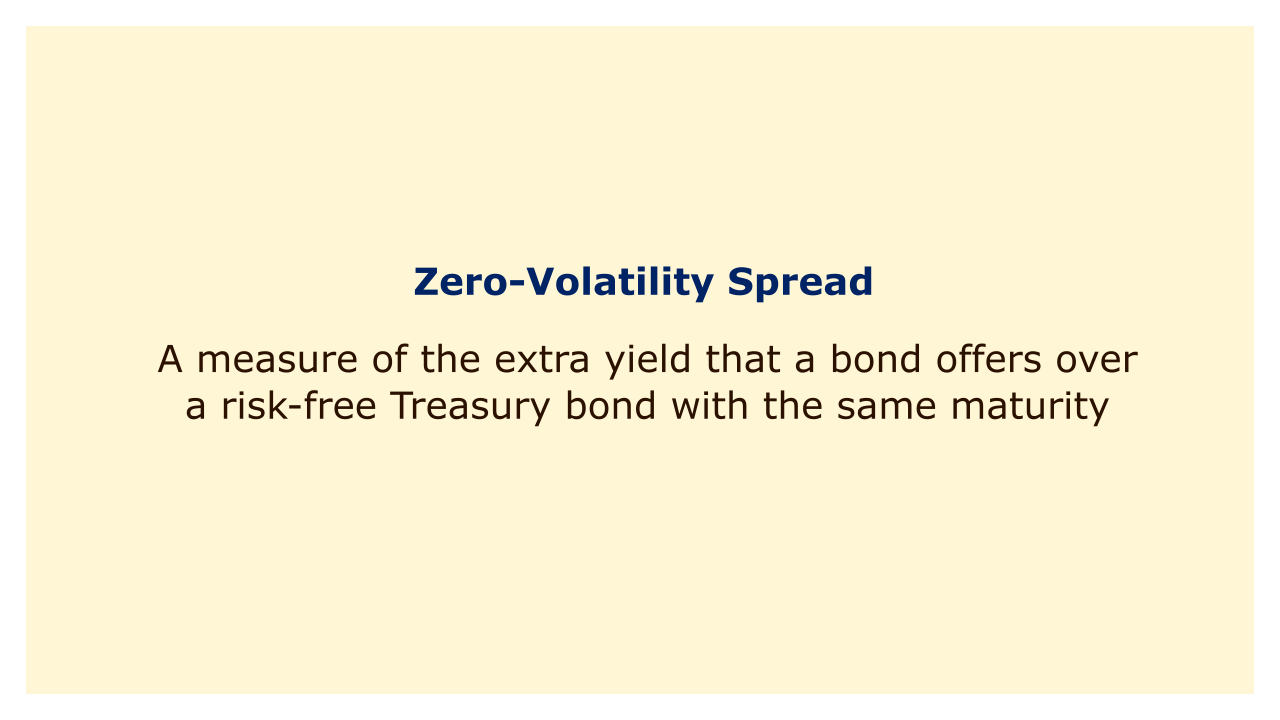Image: Moneybestpal.com

### The Zero-Volatility Spread (Z-spread) is a measure of the extra yield that a bond offers over a risk-free Treasury bond with the same maturity. Due to the fact that it is unaffected by changes in interest rates, it is often referred to as the static spread.

A bond's credit risk, liquidity risk, and embedded options are all represented by the Z-spread. In order to bring the present value of the bond's cash flows into line with its market price, it is computed by adding a fixed spread to each point on the Treasury yield curve (or Treasury spot rate curve).

Comparing bonds with various maturities, coupons, and credit ratings is helpful when using the Z-spread. It can also serve as a benchmark for the pricing of credit default swaps (CDS), which are agreements that guard against a bond issuer's default.

#### How to calculate the Z-spread?

To calculate the Z-spread, you need to know the following information:
• The current market price of the bond (including accrued interest)
• The coupon payments and maturity date of the bond
• The Treasury spot rates for each relevant maturity

The formula for calculating the Z-spread is:

P = C1 / (1 + (r1 + Z) / 2) ^ (2n) + C2 / (1 + (r2 + Z) / 2) ^ (2n) + ... + Cn / (1 + (rn + Z) / 2) ^ (2n)

where:
• P = Current market price of the bond
• Cx = Bond coupon payment at time x
• rx = Treasury spot rate at time x
• n = Relevant time period

The Z-spread can be solved by using an iterative process, such as trial and error or a solver function in Excel.

#### Example

Consider the case where you need to determine the Z-spread for a corporate bond with a 5-year maturity, a 6% annual yield, and a \$1,000 face value. Right now, the bond is worth \$1020. Spot rates for the Treasury are 1%, 1.5%, 2%, 2.5%, and 3% for the first, second, third, fourth, and fifth years, respectively.

Using the formula above, we can set up an equation as follows:

1020 = 30 / (1 + (0.01 + Z) / 2) ^ (2 * 1) + 30 / (1 + (0.015 + Z) / 2) ^ (2 * 2) + 30 / (1 + (0.02 + Z) / 2) ^ (2 * 3) + 30 / (1 + (0.025 + Z) / 2) ^ (2 * 4) + 1030 / (1 + (0.03 + Z) / 2) ^ (2 * 5)

We may determine that the value of Z that satisfies this equation is roughly 0.0128 or 128 basis points by utilizing trial and error or an Excel solver feature.

This indicates that the yield offered by the corporate bond is 128 basis points greater at each point of maturity than the yield offered by the Treasury yield curve. The increased risks of investing in corporate bonds are offset by this higher yield.
Tags